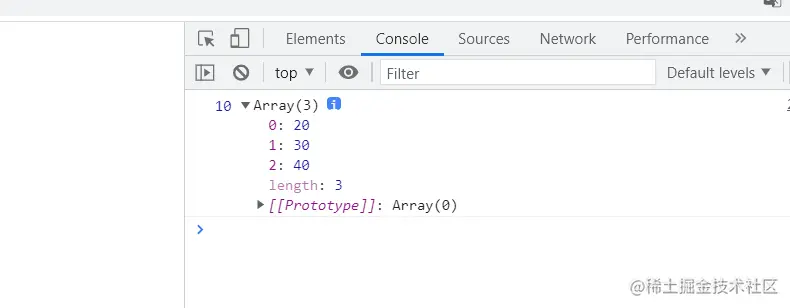## Deconstruction assignment in ES6

Colorful clouds 2021-08-09 15:48:02
deconstruction assignment es6 es

## ES6 Medium “ Deconstruct assignment ”

• Mainly for objects and arrays , The left side defines a structure similar to the right side value , Declare a few variables like this , Quick access to every part of the information .

### Array deconstruction assignment

`````` let arr = [10, 20, 30, 40];
let [x, y] = arr; // Only two variables are written , Get the first two
console.log(x, y); //10 20
Copy code ``````

The result is :`````` // “...” Remainder operator : Put aside x outside , Every item in the remaining array gets , Store in y in (y Is a new array )
let arr = [10, 20, 30, 40];
let [x, ...y] = arr;
console.log(x, y); //10 [20,30,40]
Copy code ``````

The result is ：`````` let arr = [10, 20, 30, 40];
let [, , x, y] = arr; // Only get the last two 30,40
console.log(x, y); //30 40
Copy code ``````

The result is ：`````` let arr = [10, 20, 30, 40];
let [, , , x, y = 0] = arr;
console.log(x, y); //40 0 “y=0” If it doesn't exist y This one , We assign it a default value , Otherwise it would be undefined Of
Copy code ``````

The result is ：demand : Variable exchange value ,a and b Exchange value The first method ：

`````` let a = 10;
let b = 20;
let c = a;
a = b;
b = c;
console.log(a, b); //20 10
Copy code ``````

The result is ：The second method is :

`````` let a = 10;
let b = 20;
a = a + b; //30
b = a - b; //10 a-b This is the original a Value It's equivalent to putting the original a Put the value of into b It's in
a = a - b; //20 a-b This is the original b Value It's equivalent to putting the original b Put the value of into a It's in
console.log(a, b);
Copy code ``````

The result is ：`````` let a = 10;
let b = 20;
[b, a] = [a, b]; // Build an array on the right [10,20]
console.log(a, b);
Copy code ``````

The result is ：### Object's deconstruction assignment

`````` let obj = {
name: ' The clouds ',
age: 11,
teacher: ' The clouds ',
0: 100
};
// Declared by default “ Variable ” Need and “ Property name ” bring into correspondence with , In this way, the object can get the value of the specified member
let {
name,
age
} = obj; // Declare variables name,age take obj in name,age Value .
console.log(name, age); //=>obj.name,obj.age “ The clouds ” ,11
Copy code ``````

The result is :Want to declare a variable x Come and get it obj in name Value

`````` let obj = {
name: ' The clouds ',
age: 11,
teacher: ' The clouds ',
0: 100
};
//let {x} = obj;
//console.log(x); //obj.x undefined
// If a member of an object (name) The variable corresponding to the name of has been declared , In order to prevent error reporting , To assign a new variable x Come and get it obj in name Value .
let name = 'xx';
let {
name: x // A colon means to declare a variable x, take obj in name The value of this member
} = obj;
console.log(x); //“ The clouds ”, Make a statement x The variable of , Let it is equal to the obj.name,
“ Renaming during deconstruction : Declare a variable at will , Gets the value of the specified member in the object ”
Copy code ``````

The result is ：How to make obj Middle number 0 This property name is deconstructed , Numbers cannot be used directly as variable names ,

• For numeric attribute names , We can rename a new variable to receive the value
`````` let obj = {
name: ' The clouds ',
age: 11,
teacher: ' The clouds ',
0: 100
};
let {
0: x
} = obj;
console.log(x); //=>100
Copy code ``````

The result is ：demand : Get... In the current data class 、 full name 、 Math scores The value of the three terms , String "xx Class xx Students' math scores :xx"

• The first method ： Get a little
`````` let data = [
1001,
' elite A class ',
{
name: ' The clouds ',
age: 25,
score: [98, 100, 89] // Chinese language and literature mathematics English
}
];
let className = data; // Get to class
let baseInfo = data; // get data The third one
let name = baseInfo.name; // get baseInfo Medium name
let score = baseInfo.score; // get baseInfo Medium score
let math = score; // get score The second math achievement in
console.log(`\${className} Of \${name} Students' math scores : \${math} branch `);
Copy code ``````

The result is ：• The second method ： Assign values with deconstruction
`````` let data = [1001,' elite A class ',
{
name: ' The clouds ',
age: 25,
score: [98, 100, 89] // Chinese language and literature mathematics English
}
];
let [, className,
{
name,
score: [, math] // Defining variables math Come and get it score The second value of the array
}] = data;
console.log(`\${className} Of \${name} Students' math scores : \${math} branch `);
Copy code ``````

The result is ：https://qdmana.com/2021/08/20210809153452127d.html